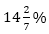Here, we are providing free crash course for LIC AAO Mains as there is left not enough time to deal in details. The questions asked in the quantitative aptitude section are calculative and very time-consuming. But once dealt with proper strategy, speed, and accuracy, this section can get you the maximum marks in the examination. Following is the Quantitative Aptitude quiz to help you practice with the best of latest pattern questions.

Directions (1-5): Table given below shows five types of article sold by a seller and selling price of each article. There is also a pie chart which shows the percentage distribution of these five articles (except article D), sold by the seller. Distribution for article D is given in absolute value

Q1. Total amount got by selling article A is what percent more the total amount got by selling article B?

Q2. What is the average amount got by selling article B, C and E together?
11700
12560
10700
12500
10520

Q3. Suppose the seller wanted to sell another article named F whose total selling price ismore than the total selling price of article C and no. of article of F is equal to the no. of article of type E. Then find the difference between price of each article of F and that of each article B ?
35
45
60
50
70

Q4. If the seller sells 2/5th of number of article of type C at 40% more than the price of each article of type B and sells the remaining article of type C at 3/5 th of price of each article of type A. Then find the difference between total new price of article of type C and the average of total price of article of type B and D?
2645
3246
3356
3646
3426

Q5. Find the ratio of average total selling price of article A and E together to the average of total selling price of article if B and C together?
32 : 17
17 : 32
32 : 19
5 : 3
33 : 17

Q6. B is 25% less efficient than A. A start working alone and work for X day and remaining work completed by B in (X + 7.5) days. Work done by A is equal to work done by B. C complete the whole work in half time in which B alone will complete the whole work , then find in how many days all three will complete the work together ?

Q7. Veer invested Rs. 21000 in a scheme on compound interest, which offered interest at the ratefor first year,of second year andfor third year. Then find how much interest will Veer get after three year on his principle ?
7500Rs.
9000Rs.
8000Rs.
8500Rs.
9500Rs.

Q8. Adda247 publication sold a puzzle book in Rs. 475 and made profit of 25% on CP and sold a DI book in Rs. 575 and made a profit of 15% on CP. If publication sold puzzle book in Rs. 360, then find what price should DI book will be sold to made a profit of 30% on both the books ?
Rs.784
Rs.874
Rs.847
Rs.748
Rs.478

Q9. A jar contains mixture of Rum and Vodka in the ratio of 4 : 1, when 10ℓ of mixture taken out and same amount of Vodka poured into jar the ratio becomes 2 : 3 then find how many liters of Rum was contained in Jar initially ?
10 l
12 l
14 l
16 l
18 l

Q10. A shopkeeper cheat both its customer and wholeseller. He take 200 gram more in buying and sells 100 gram less per kg. If selling price is 20% above the cost price then find his actual profit percent.

Directions (11-15): What will come in the place of the question mark (?) in the following number series?

Q11. 7, 16, 21, 70, 79, ?
200
121
198
90
151

Q12. 10395, 6930, 2772, 792, 176, ?
64
16
32
8
128

Q13. 7, 17, 38, 81, 168, ?
336
343
340
320
341

Q14. 21, 32, 17, 38, 13, 44, ?
7
11
10
13
9

Q15. 35, ? , 105, 160, 231, 320
63
64
55
58
75

You May also like to Read: